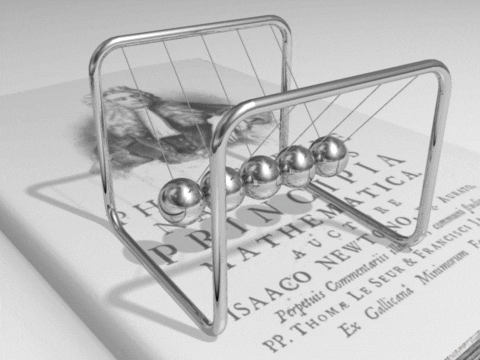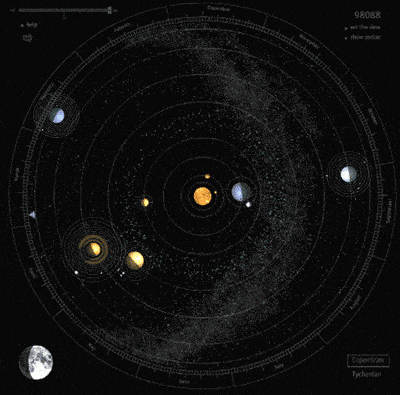Objectives:

• You should be able to calculate the amount of energy associated with a moving object
• The kinetic energy of a moving object can be calculated using the equation;$E_{k} = 0.5 \times m \times v^{2}$

Kinetic energy is the energy of motion and any object that is moving will have some. It doesn’t matter whether the object is travelling upwards, downwards, left or right, the pure fact that it is moving means it has kinetic energy.

The kinetic energy of an object is governed by two factors, the mass of an object and the speed that it is travelling at. The equation for kinetic energy that links these quantities is:$E_{k} = 0.5 m v^{2}$

where;$E_{k}$ is the kinetic energy of the object, measure in joules,$J$$m$ is the mass of the object, measured in kilograms,$kg$$v$ is the velocity of the object, measured in metres per second,$m/s$This equation tells us that for a given mass, the kinetic energy is proportional to the square of the speed of the object:$E_{k} \propto v^{2}$

What this means is that if you were to speed up so that your speed is double what it was originally, then you kinetic energy would quadruple (this assume that your mass remains constant):$E_{k} \propto (2 \times v)^{2}$$E_{k} \propto 4 \times v^{2}$$v^{2} \rightarrow$ multiplies by$4$
therefore:$E_{k} \rightarrow$ multiplies by$4$

For an object at a constant speed, the kinetic energy is proportional to the mass:$E_{k} \propto m$

What this means is that if you were to double your mass then your kinetic energy would double too (assuming your speed did not change):$E_{k} \propto (2 \times m)$$m \rightarrow$ multiples by$2$
therefore:$E_{k} \rightarrow$ multiplies by$2$

This last statement is not used as frequently because it is much more likely that the speed of an object changes rather than the mass.Energy is a pivotable concept in physics because of the statement that energy is always conserved, in other words it cannot be created nor destroyed. Kinetic energy is therefore a very significant type of energy (and so is this equation) because anything that is moving has an amount of kinetic energy that can be calculate using this equation – from a single particle to the motion of planets orbiting the sun.Examples of using this equation

1.A rugby ball of$1.2 \ kg$ is thrown with a speed of of$24 \ m/s$, how much kinetic energy does it have?
2. How much kinetic energy does a bee have after collecting its daily take of honey therefore having a total mass of$56 \ g$ and is travelling at a speed of$1.7 \ m/s$?
3. Continuing on from question 3: If the bees kinetic energy were to double, what must the new speed of the bee be?
4.Determine how much kinetic energy a$230 \ kg$ motorcycle has when the driver of mass of$80 \ kg$ is riding it at a speed of$34 \ m/s$

Solutions

1.$m = 1.2 \ kg$$v = 24 \ m/s$$E_{k} = 0.5 m v^{2} = 0.5 \times 1.2 \times 24^{2}$$E_{k} = 345.6 \ J$
2.$m = 56 \ g = 0.056 \ kg$$v = 1.7 \ m/s$$E_{k} = 0.5 m v^{2} = 0.5 \times 0.056 \times 1.7^{2}$$E_{k} = 0.081 \ J$
3.$E_{k} = 0.081 \ J$$m = 56 \ g = 0.056 \ kg$ (the mass has not changed)$E_{k} = 0.5 m v^{2} \rightarrow 0.081 = 0.5 \times 0.056 \times v^{2}$$2.89 = v^{2}$$v = 1.7 \ m/s$
4.$m = (230 + 80) \ kg = 310 \ kg$ (total mass is the rider and the bike)$v = 34 \ m/s$$E_{k} = 0.5 m v^{2} = 0.5 \times 310 \times 34^{2}$$E_{k} = 179,180 \ J = 179 \ kJ$

Where doe the equation for kinetic energy come from?